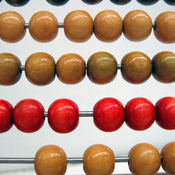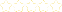• > Mark Flannery
• > MATH 1050 - Mathematical Foundations 1
+# MATH 1050 - Mathematical Foundations 1

Owner: Mark Flannery
##### Description:
This is a collection of Sophia Learning Packets that we will be using in Math 1050. Math 1050 is the first of a two-course sequence focusing on concepts and models involved with numeration systems, whole numbers, fractions, decimals, integers, real numbers, and introductory computer applications. Emphasis on mathematical reasoning, estimation, and problem solving.

### Your Quiz Points in this Playlist

Total Possible
0
3 Tutorials in this Playlist

#### Volume of a cylinder (a.k.a. Morton Salt Girl)

Author: Christopher Danielson
Rating:(7)

#### Ordpa number system basics

Author: Christopher Danielson
Rating:(9)